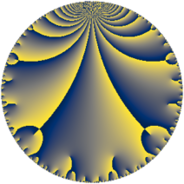Properties

 Label 207.6.aLevel $207$ Weight $6$ Character orbit 207.a Rep. character $\chi_{207}(1,\cdot)$ Character field $\Q$ Dimension $47$ Newform subspaces $9$ Sturm bound $144$ Trace bound $4$

Related objects

Defining parameters

 Level: $$N$$ $$=$$ $$207 = 3^{2} \cdot 23$$ Weight: $$k$$ $$=$$ $$6$$ Character orbit: $$[\chi]$$ $$=$$ 207.a (trivial) Character field: $$\Q$$ Newform subspaces: $$9$$ Sturm bound: $$144$$ Trace bound: $$4$$ Distinguishing $$T_p$$: $$2$$

Dimensions

The following table gives the dimensions of various subspaces of $$M_{6}(\Gamma_0(207))$$.

Total New Old
Modular forms 124 47 77
Cusp forms 116 47 69
Eisenstein series 8 0 8

The following table gives the dimensions of the cuspidal new subspaces with specified eigenvalues for the Atkin-Lehner operators and the Fricke involution.

$$3$$$$23$$FrickeDim.
$$+$$$$+$$$$+$$$$10$$
$$+$$$$-$$$$-$$$$10$$
$$-$$$$+$$$$-$$$$15$$
$$-$$$$-$$$$+$$$$12$$
Plus space$$+$$$$22$$
Minus space$$-$$$$25$$

Trace form

 $$47 q - 8 q^{2} + 800 q^{4} - 16 q^{5} + 98 q^{7} + 75 q^{8} + O(q^{10})$$ $$47 q - 8 q^{2} + 800 q^{4} - 16 q^{5} + 98 q^{7} + 75 q^{8} - 294 q^{10} - 78 q^{11} - 552 q^{13} + 1052 q^{14} + 16128 q^{16} + 2784 q^{17} - 2728 q^{19} - 3968 q^{20} - 7940 q^{22} - 1587 q^{23} + 23501 q^{25} - 8877 q^{26} + 14610 q^{28} - 2532 q^{29} - 2712 q^{31} + 6732 q^{32} + 3322 q^{34} + 8800 q^{35} + 10544 q^{37} + 46792 q^{38} - 1834 q^{40} - 13328 q^{41} - 11700 q^{43} - 7598 q^{44} + 4232 q^{46} - 65184 q^{47} + 151075 q^{49} + 87608 q^{50} - 68469 q^{52} + 104810 q^{53} + 19556 q^{55} + 56746 q^{56} - 105237 q^{58} - 5848 q^{59} - 56354 q^{61} - 38037 q^{62} + 284467 q^{64} + 95190 q^{65} - 29182 q^{67} + 2728 q^{68} + 112220 q^{70} - 94964 q^{71} + 105892 q^{73} + 34770 q^{74} - 257848 q^{76} - 36656 q^{77} - 10540 q^{79} - 159690 q^{80} - 143843 q^{82} + 62362 q^{83} + 175676 q^{85} - 7806 q^{86} - 106460 q^{88} - 354426 q^{89} - 428658 q^{91} - 50784 q^{92} + 185761 q^{94} - 47476 q^{95} - 181052 q^{97} + 325756 q^{98} + O(q^{100})$$

Decomposition of $$S_{6}^{\mathrm{new}}(\Gamma_0(207))$$ into newform subspaces

Label Dim. $$A$$ Field CM Traces A-L signs $q$-expansion
$a_{2}$ $a_{3}$ $a_{5}$ $a_{7}$ 3 23
207.6.a.a $2$ $33.199$ $$\Q(\sqrt{29})$$ None $$0$$ $$0$$ $$-94$$ $$-118$$ $-$ $+$ $$q-2\beta q^{2}+84q^{4}+(-47-\beta )q^{5}+(-59+\cdots)q^{7}+\cdots$$
207.6.a.b $3$ $33.199$ 3.3.7925.1 None $$4$$ $$0$$ $$58$$ $$-282$$ $-$ $-$ $$q+(1-\beta _{1})q^{2}+(2-6\beta _{1}+4\beta _{2})q^{4}+\cdots$$
207.6.a.c $3$ $33.199$ 3.3.5333.1 None $$8$$ $$0$$ $$56$$ $$-114$$ $-$ $+$ $$q+(3-\beta _{2})q^{2}+(9-4\beta _{1}-9\beta _{2})q^{4}+\cdots$$
207.6.a.d $4$ $33.199$ $$\mathbb{Q}[x]/(x^{4} - \cdots)$$ None $$-4$$ $$0$$ $$122$$ $$62$$ $-$ $+$ $$q+(-1-\beta _{1})q^{2}+(-12+4\beta _{1}-\beta _{2}+\cdots)q^{4}+\cdots$$
207.6.a.e $4$ $33.199$ $$\mathbb{Q}[x]/(x^{4} - \cdots)$$ None $$-4$$ $$0$$ $$-22$$ $$-62$$ $-$ $-$ $$q+(-1+\beta _{1})q^{2}+(7-\beta _{1}+\beta _{3})q^{4}+\cdots$$
207.6.a.f $5$ $33.199$ $$\mathbb{Q}[x]/(x^{5} - \cdots)$$ None $$-8$$ $$0$$ $$-94$$ $$272$$ $-$ $-$ $$q+(-2+\beta _{2})q^{2}+(24-\beta _{2}+\beta _{3})q^{4}+\cdots$$
207.6.a.g $6$ $33.199$ $$\mathbb{Q}[x]/(x^{6} - \cdots)$$ None $$-4$$ $$0$$ $$-42$$ $$300$$ $-$ $+$ $$q+(-1+\beta _{1})q^{2}+(20-2\beta _{1}+\beta _{2}+\beta _{3}+\cdots)q^{4}+\cdots$$
207.6.a.h $10$ $33.199$ $$\mathbb{Q}[x]/(x^{10} - \cdots)$$ None $$-8$$ $$0$$ $$-100$$ $$20$$ $+$ $+$ $$q+(-1+\beta _{1})q^{2}+(19-\beta _{1}+\beta _{2})q^{4}+\cdots$$
207.6.a.i $10$ $33.199$ $$\mathbb{Q}[x]/(x^{10} - \cdots)$$ None $$8$$ $$0$$ $$100$$ $$20$$ $+$ $-$ $$q+(1-\beta _{1})q^{2}+(19-\beta _{1}+\beta _{2})q^{4}+(9+\cdots)q^{5}+\cdots$$

Decomposition of $$S_{6}^{\mathrm{old}}(\Gamma_0(207))$$ into lower level spaces

$$S_{6}^{\mathrm{old}}(\Gamma_0(207)) \cong$$ $$S_{6}^{\mathrm{new}}(\Gamma_0(3))$$$$^{\oplus 4}$$$$\oplus$$$$S_{6}^{\mathrm{new}}(\Gamma_0(9))$$$$^{\oplus 2}$$$$\oplus$$$$S_{6}^{\mathrm{new}}(\Gamma_0(23))$$$$^{\oplus 3}$$$$\oplus$$$$S_{6}^{\mathrm{new}}(\Gamma_0(69))$$$$^{\oplus 2}$$